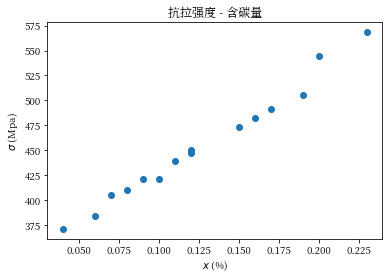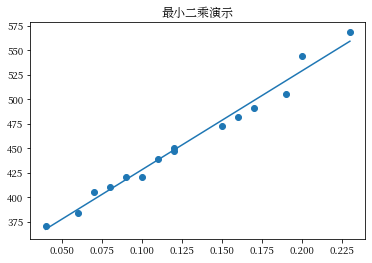# 使用 Pandoc 进行文档编辑

Pandoc 是一个标记语言转换工具，它支持非常多的语言之间的相互转换，并且，支持一种功能非常强大的 Markdown 解析功能， 也提出了对应的标准： Pandoc Markdown 1

# 在 PowerShell 中并行执行任务

Job 是一个新的 PowerShell 进程，而不是线程。

# 用 C 语言扩展 Python

Python 官方给出教程如下 1，本文总结几个要点，并介绍与 Python Poetry 2 工具相结合的方法。

# 数值计算：回归分析

In :
import numpy as np
import matplotlib.pyplot as plt
plt.rcParams["font.family"] = "Source Han Serif CN"


## 回归分析¶

• 线性：自变量的增量对应的因变量的增量是恒定的
• 非线性：自变量的增量对应的因变量的增量是不恒定的

### 一元线性回归¶

$$(x_1, y_1), (x_2, y_2), \cdots, (x_n, y_n)$$

In :
carbon = np.array([0.04, 0.06, 0.07, 0.08, 0.09, 0.10, 0.11, 0.12, 0.12, 0.15, 0.16, 0.17, 0.19, 0.20, 0.23])
sigma = np.array([
371.00,
384.00,
405.00,
410.00,
421.00,
421.00,
439.00,
447.00,
450.00,
473.00,
482.00,
491.00,
505.00,
544.00,
569.00,
])


In :
fig0 = plt.figure()
ax0.scatter(carbon, sigma)
ax0.set_title("抗拉强度 - 含碳量")
ax0.set_xlabel("$x$ (%)")
ax0.set_ylabel("$\\sigma$ (Mpa)")
fig0;$$\hat{y} = k x + b \tag{1'}$$

[a0, a1, a2, a3, ..., an]


$$\hat{y} = p_0 + p_1 x \tag{1}$$

\begin{aligned} & [y_i - \hat{y}(x_i)]^2 \\ =& [y_i - (p_0 + p_1 x_i)]^2 \end{aligned} \tag{2.1}

$$Q(p_0, p_1) = \sum_{i=1}^{N} [y_i - (p_0 + p_1 x_i)]^2 \tag{2.2}$$

### 最小二乘法¶

\newcommand{\pp}{\frac{\partial{}#1}{\partial{}#2}} \begin{aligned} \pp{Q}{p_0} &= -2 \sum_{i=1}^{N} [y_i - (p_0 + p_1 x_i)] &= 0 \\ \pp{Q}{p_1} &= -2 \sum_{i=1}^{N} [y_i - (p_0 + p_1 x_i)] x_i &= 0 \end{aligned} \tag{3.1}

$$\begin{cases} N &p_0 + (\sum_{i=1}^{N} x_i)&p_1 = \sum_{i=1}^{N} y_i \\ (\sum_{i=1}^{N} x_i) &p_0 + (\sum_{i=1}^{N} x_i^2) &p_1 = \sum_{i=1}^{N} x_i y_i \end{cases} \tag{3.2}$$



$$\begin{cases} \hat{p_0} &= \overline{y} - \hat{p_1} \overline{x} \\ \hat{p_1} &= \frac{\sum_{i=1}^{N} (x_i - \overline{x})(y_i - \overline{y})}{\sum_{i=1}^{N} (x_i - \overline{x})^2} \end{cases} \tag{3.6}$$

In :
def my_leastsq(x, y):
"""最小二乘法

:param x: 一列数据
:param y: 与 x 维度相同的一列数据
:returns: 拟合曲线的截距，斜率
"""
length = len(x)
x_avg = np.sum(x) / length
y_avg = np.sum(y) / length

xx = x - x_avg
yy = y - y_avg

p1 = np.sum(xx * yy) / np.sum(xx * xx)
p0 = y_avg - p1 * x_avg

return p0, p1

In :
_b, _k = my_leastsq(carbon, sigma)

_fig = plt.figure()
_ax.scatter(carbon, sigma)
_ax.plot(carbon, _k * carbon + _b)
_ax.set_title("最小二乘演示")
_fig;
# todo 置信度，相关系数### 一元非线性回归¶

\begin{aligned} \frac{1}{}: &y = \frac{1}{x} \to y = x', x' = 1/x \\ \ln{}: &y = e^x \to \ln{y} = x \\ \vdots \end{aligned}

# Windows PowerShell 查看端口占用

TCP 链接和 UDP 链接的查询指令是分别的两个：

Get-NetTCPConnection

Get-NetUDPEndPoint

Get-NetTCPConnection -LocalPort 8000

Get-Process -Id (Get-NetTCPConnection -LocalPort 8000).OwningProcess

# Windows 文件访问权限重置

icacls * /reset /T /C
*

/reset

/T

/C